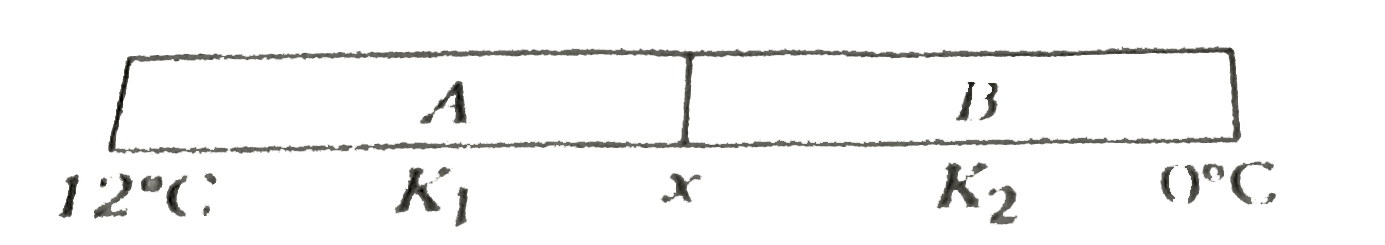# Two slabs A and B of different materials but of the same thicknesss are joined end to end to form a composite slab. The thermal conductivities of A an

185 views
in Physics
closed
Two slabs A and B of different materials but of the same thicknesss are joined end to end to form a composite slab. The thermal conductivities of A and B are K_(1) and K_(2) respectively. A steady temperature difference of 12^(@)C is maintained across the composite slab. If K_(1) = K_(2)/2, the temperature difference across slabs A is
A. 4^(@)C
B. 6^(@)C
C. 8^(@)C
D. 10^(@)C

by (90.7k points)
selected

The given equation can be shown asRate of flow of heat will be equal in both the slabs
therefore (12-x)K_(1)= K_(2) (x-0)
12-x = 2x rArr x=4^(@)C (therefore K_(1) = (K_(2)/2)
The temperature difference across slab
A = (12-x) = (12-4) = 8^(@)C